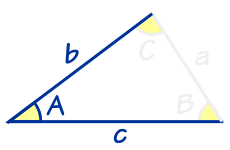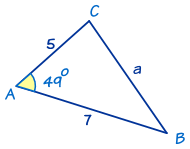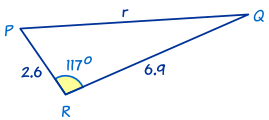# 解 SAS 三角形

"SAS" 的意思是 "(S)ide, (A)ngle, (S)ide"（英语 "Angle" 的意思是 角，"Side" 的意思是 边）"SAS" 的意思是我们知道两个边长和这两边之间的角度。

### 例 1• 角 A = 49°
• 边 b = 5
• 边 c = 7

a2 = b2 + c2 − 2bc cosA

a2 = 52 + 72 − 2 × 5 × 7 × cos(49°)
a2 = 25 + 49 − 70 × cos(49°)
a2 = 74 − 70 × 0.6560……
a2 = 74 − 45.924…… = 28.075……
a = √28.075……
a = 5.298……
a = 5.30 （保留2个小数位）

sin B / b = sin A / a

sin B / 5 = sin(49°) / 5.298……

sin B = (sin(49°) × 5) / 5.298……
sin B = 0.7122……
B = sin−1(0.7122……)
B = 45.4° （准确到一个小数位）

C = 180° − 49° − 45.4°
C = 85.6° （准确到一个小数位）

### 例 2r2 = p2 + q2 − 2pq cos R

r2 = 6.92 + 2.62 − 2 × 6.9 × 2.6 × cos(117°)
r2 = 47.61 + 6.76 − 35.88 × cos(117°)
r2 = 54.37 − 35.88 × (−0.4539……)
r2 = 54.37 + 16.289…… = 70.659……
r = √70.659……
r = 8.405…… = 8.41 （准确到2个小数位）

sin P / p = sin R / r

sin P / 6.9 = sin(117°) / 8.405……
sin P = ( sin(117°) × 6.9 ) / 8.405……
sin P = 0.7313……
P = sin−1(0.7313……)
P = 47.0° （准确到一个小数位）

Q = 180° − 117° − 47.0°
Q = 16.0° （准确到一个小数位）